# Incidence system

(diff) ← Older revision | Latest revision (diff) | Newer revision → (diff)

A familyof two setsand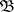with an incidence relationbetween their elements, which is written as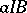for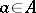,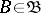. In this case one says that the elementis incident with, or thatis incident with. The concept of an incidence system is introduced with the purpose of using the language of geometry in the study of general combinatorial existence and construction problems; the incidence relation is ascribed certain properties that lead to some or other combinatorial configurations.

An example of incidence systems used in combinatorics are (finite) geometries: the elements of the (finite) setsandare called, respectively, points and lines, andis a relation with properties that are usual in the theory of projective or affine geometry. Another characteristic example of incidence systems is that of block designs (cf. Block design), which are obtained by requiring that 1) eachis incident with preciselyelements of; 2) eachis incident with preciselyelements of; and 3) each pair of distinct elements ofis incident with preciselyelements of. Often a set of subsets ofis taken for; thenis simply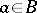.

Two incidence systems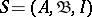and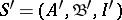are called isomorphic if there are one-to-one correspondences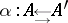and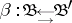such that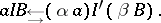If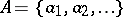andare finite sets, then the properties of the incidence systemcan be conveniently described by the incidence matrix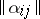, where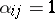if, and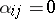otherwise. The matrixdeterminesup to an isomorphism.

How to Cite This Entry:
Incidence system. Encyclopedia of Mathematics. URL: http://encyclopediaofmath.org/index.php?title=Incidence_system&oldid=19258
This article was adapted from an original article by V.E. Tarakanov (originator), which appeared in Encyclopedia of Mathematics - ISBN 1402006098. See original article